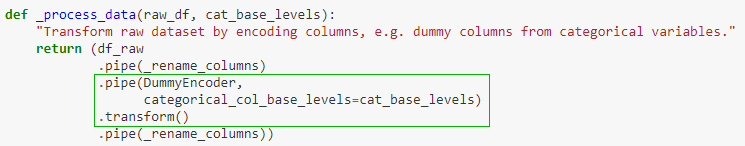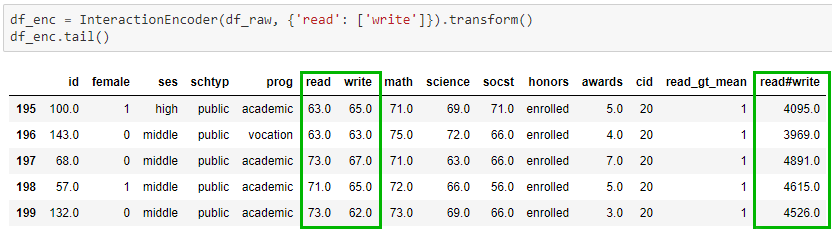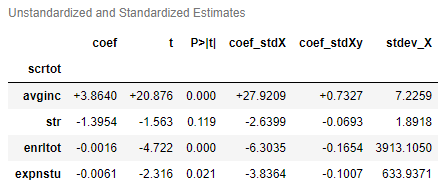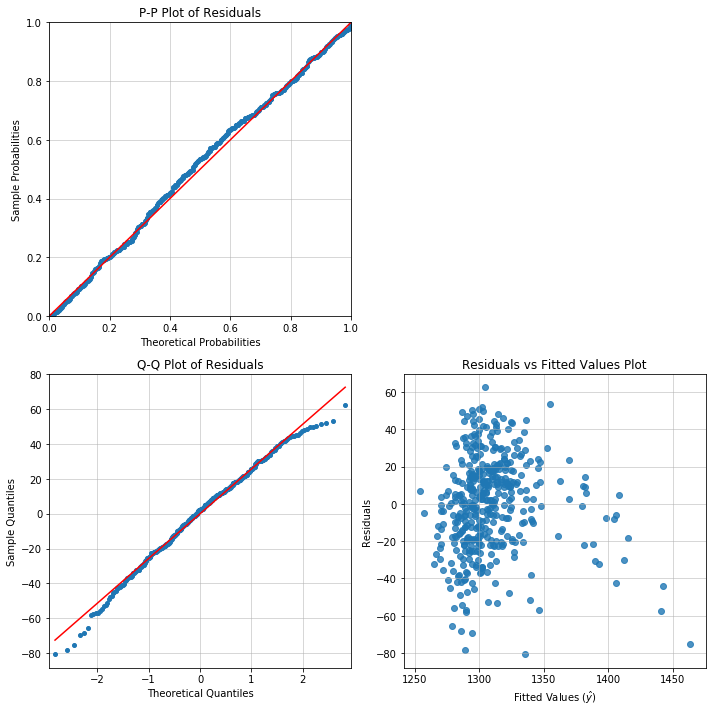These are some reasons why Appelpy adds so much more to the vanilla Python data stack:

## General principles

• Fluent interface and API design make it easier to build pipelines for modelling & data pre-processing.
• Appelpy is a more accessible Python library for Stata users, by having a simple syntax and having most functionality rely on a fitted model object, while realising the benefits of object-oriented programming.: click the badge to see a demonstration of model pipelines with Appelpy.

## Data transformation

• Useful encoders for transforming datasets, e.g.:
• `DummyEncoder`: encode dummy columns from variables based on different policies for handling NaN values.
• `InteractionEncoder`: encode columns (in a batch) for the interaction effect between two variables.
• `get_dataframe_columns_diff` method makes it easier to compare the columns between two dataframes when building pipelines for transforming data.## Exploratory data analysis (EDA)

• One method to examine all `statistical_moments` in a dataset: mean, variance, skewness and kurtosis.
• Correlation heatmap plot with triangular matrix to maximise the data–ink ratio.

## Model estimation

Model objects take on a special importance in Appelpy. More attributes and methods are available through these model objects. These are examples of what model objects contain:

• Standardized model estimates (Beta coefficients): `model.results_output_standardized`.
• Standardized `y` and `X` values.
• Access the main metrics of a model via `model.model_selection_stats`, e.g. Root MSE for OLS models.
• Odds ratio available for `Logit` models.## Model diagnostics

It's easy enough to fit a model in Statsmodels. Where Appelpy shines is the ability to do diagnostics using only the model object, instead of having to call multiple methods scattered across a library. These are some diagnostics available:

• One method for calling diagnostic plots to assess whether OLS assumptions hold in a model, e.g. `model.diagnostic_plot('rvf_plot')`. The plots are similar to commands available in Stata, but here they are accessible within the Appelpy OLS model object.
• `BadApples` class does a decomposition of influence analysis into three parts: leverage, outlier and influence measures. The leverage vs residuals squared plot (similar to Stata's `lvr2plot`) can also be called from an instance of the class.
• Identify extreme observations in a model based on common heuristics.
• Perform diagnostics not implemented in the main Python libraries, e.g. studentized Breusch–Pagan test of heteroskedasticity.
• Joint hypothesis testing, using built-in Python data structures (lists & dicts).## Model usage

• Model prediction methods have the option to return predictions only for observations that are 'within sample'.